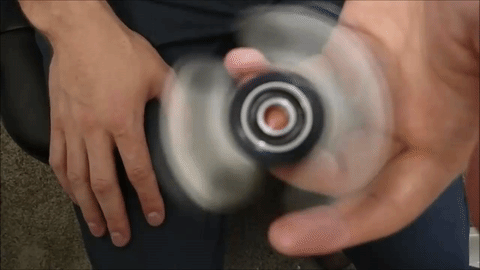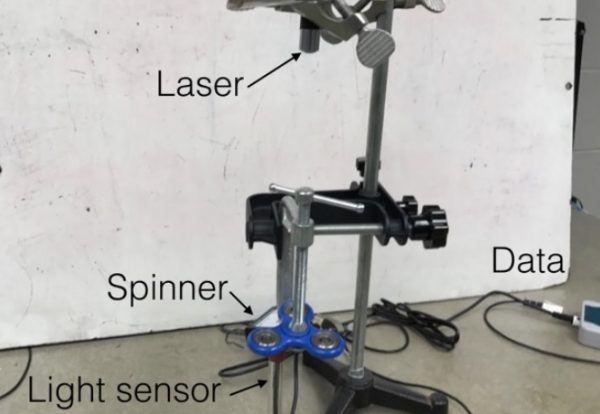The physics of fidget spinnersWired's Rhett Allain built a rig with a laser and light sensor to study fidget spinner physics and determine how long it will spin based on the starting angular velocity. Allain's article will make a great teachable moment for my kids, as in I'll ask them to read it and explain it to me. From Wired:

If I know the starting angular speed and I assume a final angular speed of zero radians per second, I can calculate the spin time:All I need is the angular acceleration—assuming it remains constant as the spinner slows. I could calculate the angular acceleration based on the change in angular velocity, but this isn’t so simple to measure. The spinner moves too quickly to get a good video of its motion, so I will use a laser in a rig I built to measure the change in the angular velocity.Basically, the laser shines down onto a light sensor. As the spinner spins, it occasionally blocks the sensor, interrupting the laser. By measuring the values from the light sensor, I determine the spin rate. But this creates a couple of problems. First, the light change rate and the rotation rate differ because the three “lobes” in the spinner create multiple openings during each rotation. Second, the spinner will spin for a significant amount of time such that it would be difficult to analyze it all at once...

Now for the fun trick. Instead of looking at a giant plot of light vs. time (the full data is over 2 minutes), I will plot the Fourier transform of this data. What does that even mean? Well, suppose this data is made of many different trigonometry functions (like sine and cosine) with different frequencies (which it essentially is). These trig functions have different amplitudes. So, the Fourier transform shows the amplitude of different frequencies, so you can determine the frequency of oscillation.